Author: Oscar Cronquist Article last updated on May 05, 2022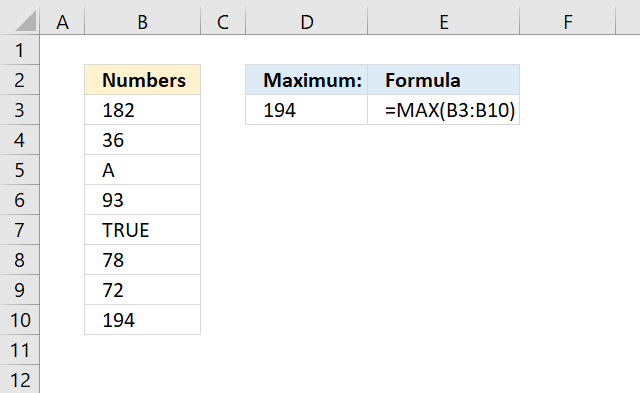The MAX function calculates the largest number in a cell range. The formula in cell D3 extracts the highest number from cell range B3:B10, see picture above.

Formula in cell D3:

=MAX(B3:B10)

## 1. MAX Function Syntax

MAX(number1, [number2], ...)

## 2. MAX Function Arguments

 Argument Text number1 You need at least one argument. Required. [number2] Up to 254 arguments are possible. Optional.

Arguments can be constants, named ranges, cell references, and arrays. The MAX function ignores text and boolean values, however not errors.

## 3. Excel function not working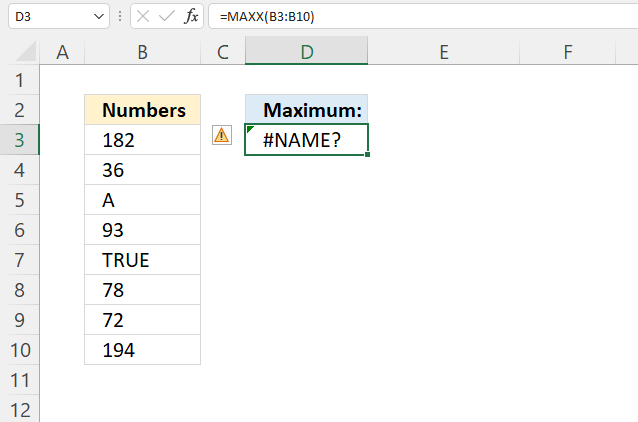A #NAME? error is most often the result of a misspelled function. The image above shows the MAX function in cell D3, however, it returns a #NAME? error.

### 3.1 Error in data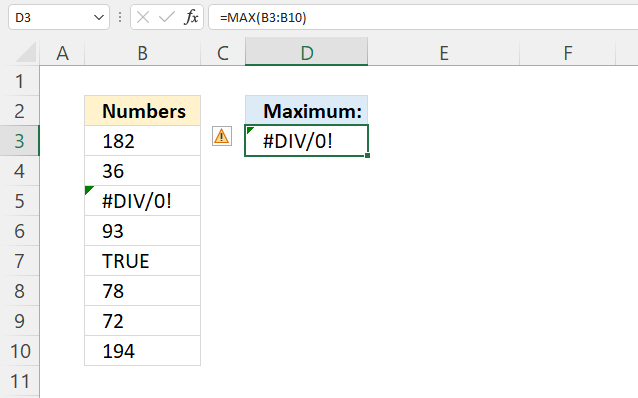The MAX function can't handle error values in your data. The first error parsed is returned. The image above shows a #DIV/0! error in cell D3, data in cell B3:B10 contains a #DIV/0 error in cell B5.

## 4. Max function based on a condition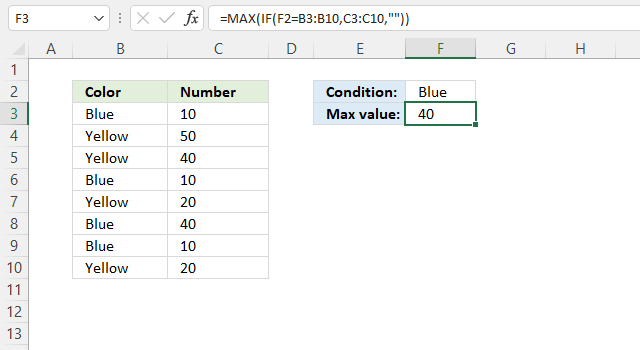Array formula in cell F3:

=MAX(IF(F2=B3:B10, C3:C10, ""))

### 4.1 Explaining formula in cell F3

#### Step 1 - Compare values

The equal sign lets you compare value to value, the result is a boolean value TRUE or FALSE.

F2=B3:B10

becomes

"Blue"={"Blue"; "Yellow"; "Yellow"; "Blue"; "Yellow"; "Blue"; "Blue"; "Yellow"}

and returns

{TRUE; FALSE; FALSE; TRUE; FALSE; TRUE; TRUE; FALSE}.

#### Step 2 - Evaluate IF function

The IF function returns one value if the logical test is TRUE and another value if the logical test is FALSE.

IF(logical_test, [value_if_true], [value_if_false])

IF(F2=B3:B10, C3:C10, "")

becomes

IF({TRUE; FALSE; FALSE; TRUE; FALSE; TRUE; TRUE; FALSE}, C3:C10, "")

becomes

IF({TRUE; FALSE; FALSE; TRUE; FALSE; TRUE; TRUE; FALSE}, {10; 50; 40; 10; 20; 40; 10; 20}, "")

and returns

{10; ""; ""; 10; ""; 40; 10; ""}.

#### Step 3 - Return max number

The MAX function calculates the largest number in a cell range.

MAX(number1, [number2], ...)

MAX(IF(F2=B3:B10, C3:C10, ""))

becomes

MAX({10; ""; ""; 10; ""; 40; 10; ""})

and returns 40 in cell F3.

## 5. Get the largest number based on multiple conditions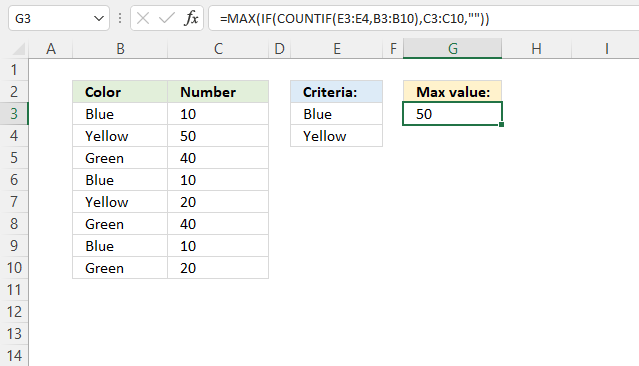The image above shows a formula in cell G3 that extracts the largest number if the adjacent value is equal to the criteria specified in cell E3:E4.

Array formula in cell G3:

=MAX(IF(COUNTIF(E3:E4,B3:B10),C3:C10,""))

### 5.1 Explaining formula in cell F3

#### Step 1 - Identify cells equal to any of the criteria

The COUNTIF function calculates the number of cells equal to a condition.

COUNTIF(rangecriteria)

COUNTIF(E3:E4,B3:B10)

becomes

COUNTIF({"Blue"; "Yellow"},{"Blue"; "Yellow"; "Green"; "Blue"; "Yellow"; "Green"; "Blue"; "Green"})

and returns {1; 1; 0; 1; 1; 0; 1; 0}. 1 means that the value in that position is equal to one of the conditions, this means that the position in the array is important in order to extract correct numbers.

#### Step 2 - Replace 1 with the corresponding number

The IF function returns one value if the logical test is TRUE and another value if the logical test is FALSE.

IF(logical_test, [value_if_true], [value_if_false])

IF(COUNTIF(E3:E4, B3:B10), C3:C10, "")

becomes

IF({1; 1; 0; 1; 1; 0; 1; 0}, C3:C10, "")

becomes

IF({1; 1; 0; 1; 1; 0; 1; 0}, {10; 50; 40; 10; 20; 40; 10; 20}, "")

and returns {10; 50; ""; 10; 20; ""; 10; ""}.

#### Step 3 - Return the largest number in the array

The MAX function calculates the largest number in a cell range.

MAX(number1, [number2], ...)

MAX(IF(COUNTIF(E3:E4, B3:B10), C3:C10, ""))

becomes

MAX({10; 50; ""; 10; 20; ""; 10; ""})

and returns 50 in cell G3.

## 6. Max function for textThe following section explains how to filter the largest value based on the number of characters in a cell, if you are looking for the most repeated value go to section 6.2 below.

Array formula in cell F3:

=INDEX(B3:B12, MATCH(MAX(LEN(B3:B12)), LEN(B3:B12), 0))

### 6.1 Explaining formula in cell F3

#### Step 1 - Count characters

The LEN function counts the number of characters in a cell.

LEN(B3:B12)

becomes

LEN({"Emphasis"; "Basil"; "Boolean"; "Doors"; "Gift"; "Historian"; "Integration"; "Pension"; "Poverty"; "Yogurt"})

and returns {8; 5; 7; 5; 4; 9; 11; 7; 7; 6}.

#### Step 2 - Get largest character count

The MAX function calculates the largest number in a cell range.

MAX(number1, [number2], ...)

MAX(LEN(B3:B12))

becomes

MAX({8; 5; 7; 5; 4; 9; 11; 7; 7; 6})

and returns 11.

#### Step 3 - Find the position of a cell containing the largest number of characters

The MATCH function returns the relative position of an item in an array or cell reference that matches a specified value in a specific order.

MATCH(lookup_value, lookup_array, [match_type])

MATCH(MAX(LEN(B3:B12)), LEN(B3:B12), 0)

becomes

MATCH(11, {8; 5; 7; 5; 4; 9; 11; 7; 7; 6}, 0)

and returns 7.

#### Step 4 - Get value based on position

The INDEX function returns a value from a cell range, you specify which value based on a row and column number.

INDEX(B3:B12, MATCH(MAX(LEN(B3:B12)), LEN(B3:B12), 0))

becomes

INDEX(B3:B12, 7)

becomes

INDEX({"Emphasis"; "Basil"; "Boolean"; "Death"; "Gift"; "Historian"; "Integration"; "Pension"; "Poverty"; "Yogurt"}, 7)

and returns "Integration" in cell D3.

### 6.2 Get most frequent value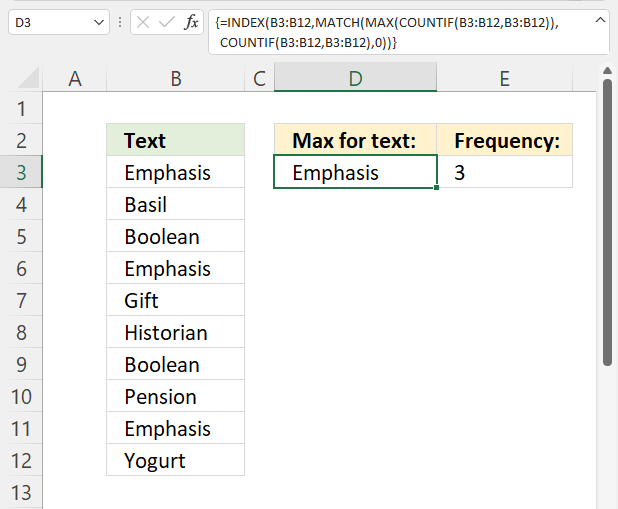The formula below extracts the most repeated text value in cell range B3:B12.

Formula in cell D3:

=INDEX(B3:B12, MATCH(MAX(COUNTIF(B3:B12, B3:B12)), COUNTIF(B3:B12, B3:B12), 0))

Use the MODE function if you are working with numbers.

#### 6.2.1 Explaining formula in cell D3

Step 1 - Count value frequency

The COUNTIF function calculates the number of cells equal to a condition.

COUNTIF(rangecriteria)

COUNTIF(B3:B12, B3:B12)

becomes

COUNTIF({"Emphasis"; "Basil"; "Boolean"; "Emphasis"; "Gift"; "Historian"; "Boolean"; "Pension"; "Emphasis"; "Yogurt"},{"Emphasis"; "Basil"; "Boolean"; "Emphasis"; "Gift"; "Historian"; "Boolean"; "Pension"; "Emphasis"; "Yogurt"})

and returns

{3; 1; 2; 3; 1; 1; 2; 1; 3; 1}.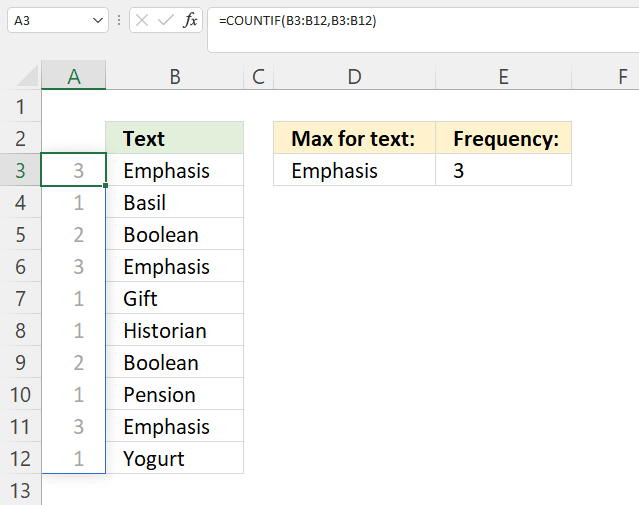The array is displayed in cell range A3:A12, each number corresponds on the same row to a value in B3:B12. Cell value in B3 is found three times in B3:B12, the array shows 3 in cell A3.

Step 2 - Get most frequent value

The MAX function calculates the largest number in a cell range.

MAX(number1, [number2], ...)

MAX(COUNTIF(B3:B12, B3:B12))

becomes

MAX({3; 1; 2; 3; 1; 1; 2; 1; 3; 1})

and returns 3.

Step 3 - Find position of most frequent value

The MATCH function returns the relative position of an item in an array or cell reference that matches a specified value in a specific order.

MATCH(lookup_value, lookup_array, [match_type])

MATCH(MAX(COUNTIF(B3:B12, B3:B12)), COUNTIF(B3:B12, B3:B12), 0)

becomes

MATCH(3, COUNTIF(B3:B12, B3:B12), 0)

becomes

MATCH(3, {3; 1; 2; 3; 1; 1; 2; 1; 3; 1}, 0)

and returns 1.

Step 4 - Get most frequent value

The INDEX function returns a value from a cell range, you specify which value based on a row and column number (optional).

INDEX(reference, row, column)

INDEX(B3:B12, MATCH(MAX(COUNTIF(B3:B12, B3:B12)), COUNTIF(B3:B12, B3:B12), 0))

becomes

INDEX(B3:B12, 1)

becomes

INDEX({"Emphasis"; "Basil"; "Boolean"; "Emphasis"; "Gift"; "Historian"; "Boolean"; "Pension"; "Emphasis"; "Yogurt"}, 1)

and returns "Emphasis".

## 7. MAX function ignore #N/A error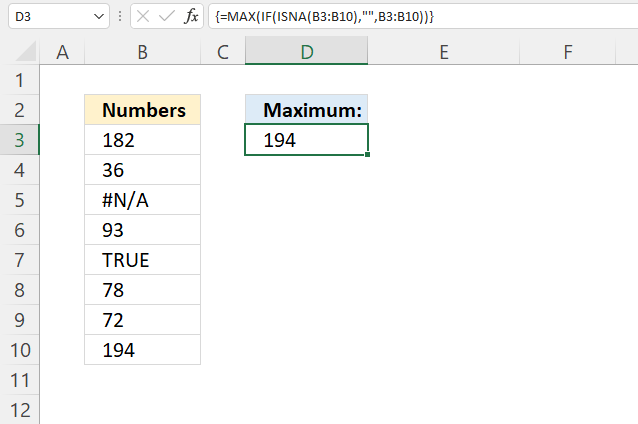This formula returns the largest number from a given cell range ignoring #N/A errors.

Array formula in cell D3:

=MAX(IF(ISNA(B3:B10), "", B3:B10))

### 7.1 Explaining formula

#### Step 1 - Identify #N/A errors

The ISNA function returns TRUE if a value is a #N/A error.

ISNA(value)

ISNA(B3:B10)

becomes

ISNA({182; 36; #N/A; 93; TRUE; 78; 72; 194})

and returns

{FALSE; FALSE; TRUE; FALSE; FALSE; FALSE; FALSE; FALSE}.

#### Step 2 - Replace #N/A errors with a blank

The IF function returns one value if the logical test is TRUE and another value if the logical test is FALSE.

IF(logical_test, [value_if_true], [value_if_false])

IF(ISNA(B3:B10), "", B3:B10)

becomes

IF({FALSE; FALSE; TRUE; FALSE; FALSE; FALSE; FALSE; FALSE}, "", {182; 36; #N/A; 93; TRUE; 78; 72; 194})

and returns

{182; 36; ""; 93; TRUE; 78; 72; 194}.

The N/A error is replaced with a blank in the array above.

#### Step 3 - Extract the largest number ignoring blanks and text values

MAX(IF(ISNA(B3:B10), "", B3:B10))

becomes

MAX({182; 36; ""; 93; TRUE; 78; 72; 194})

and returns 194.

## 8. MAX function second-highest number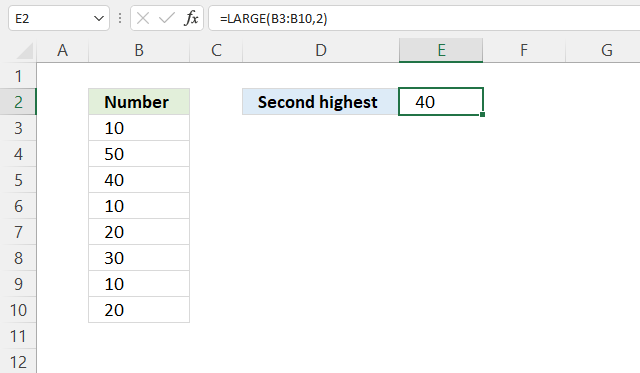The image above demonstrates a formula in cell E2 that extracts the second largest number from cell range B3:B10.

Formula in cell E2:

=LARGE(B3:B10,2)

The LARGE function extracts the k-th largest number in a cell range or array.

LARGE(array, k)

Argument k becomes 2 if we want to extract the second-largest number.

The formula returns the largest number if it has a duplicate. Consider these numbers, 3, 2, and 3. The largest number is three, the second-largest number is also three. This is because number three has a duplicate.

## 9. MAX function second-highest number no duplicates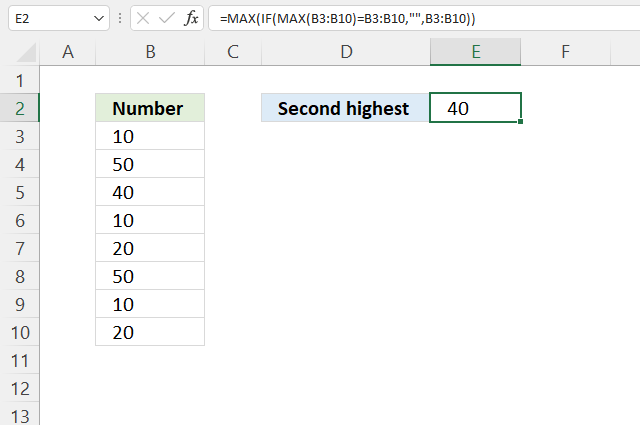The image above demonstrates a formula in cell E2 that extracts the second largest number, duplicate numbers are ignored.

For example, both cells B4 and B8 contain the number 50. The LARGE function returns the second largest number considering duplicate numbers as well. The following function ignores duplicates returnning 40 in cell E4.

Formula in cell E2:

=MAX(IF(MAX(B3:B10)=B3:B10, "", B3:B10))

### 9.1 Explaining formula in cell E2

#### Step 1 - Find the largest number

The MAX function calculates the largest number in a cell range.

MAX(number1, [number2], ...)

MAX(B3:B10)

becomes

MAX({10; 50; 40; 10; 20; 50; 10; 20})

and returns 50.

#### Step 2 - Find the largest number

The equal sign lets you compare value to value, the result is a boolean value TRUE or FALSE.

MAX(B3:B10)=B3:B10

becomes

50={10; 50; 40; 10; 20; 50; 10; 20}

and returns

{FALSE; TRUE; FALSE; FALSE; FALSE; TRUE; FALSE; FALSE}.

#### Step 3 - Replace boolean value TRUE with corresponding numbers

The IF function returns one value if the logical test is TRUE and another value if the logical test is FALSE.

IF(logical_test, [value_if_true], [value_if_false])

IF(MAX(B3:B10)=B3:B10, "", B3:B10)

becomes

IF({FALSE; TRUE; FALSE; FALSE; FALSE; TRUE; FALSE; FALSE}, "", B3:B10)

becomes

IF({FALSE; TRUE; FALSE; FALSE; FALSE; TRUE; FALSE; FALSE}, "", {10; 50; 40; 10; 20; 50; 10; 20})

and returns

{""; 50; ""; ""; ""; 50; ""; ""}.

#### Step 4 - Extract maximum number from array

MAX(IF(MAX(B3:B10)=B3:B10, "", B3:B10))

becomes

MAX({""; 50; ""; ""; ""; 50; ""; ""})

and returns 50.

## 10. MAX function second-highest number based on a condition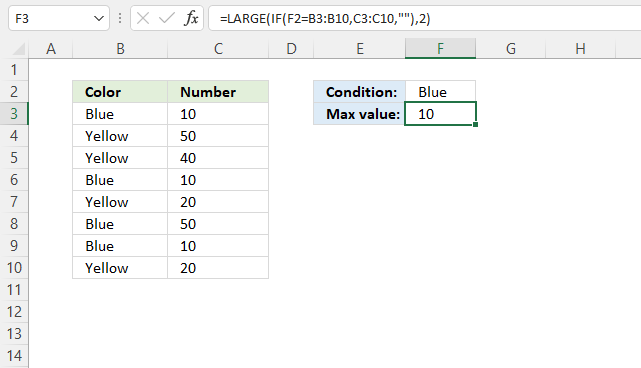Formula in cell F3:

=LARGE(IF(F2=B3:B10,C3:C10,""),2)

### 10.1 Explaining formula

#### Step 1 - Identify values equal to condition

The equal sign lets you compare value to value, in this case, value to array. The result is a boolean value TRUE or FALSE.

F2=B3:B10

becomes

"Blue"={"Blue"; "Yellow"; "Yellow"; "Blue"; "Yellow"; "Blue"; "Blue"; "Yellow"}

and returns

{TRUE; FALSE; FALSE; TRUE; FALSE; TRUE; TRUE; FALSE}.

#### Step 2 - Replace TRUE with corresponding number on the same row

The IF function returns one value if the logical test is TRUE and another value if the logical test is FALSE.

IF(logical_test, [value_if_true], [value_if_false])

IF(F2=B3:B10, C3:C10, "")

becomes

IF({TRUE; FALSE; FALSE; TRUE; FALSE; TRUE; TRUE; FALSE}, {10; 50; 40; 10; 20; 50; 10; 20}, "")

and returns

{10; ""; ""; 10; ""; 50; 10; ""}.

#### Step 3 - Extract second largest number from the array

The LARGE function extracts the k-th largest number in a cell range or array.

LARGE(array, k)

LARGE(IF(F2=B3:B10, C3:C10, ""), 2)

becomes

LARGE({10; ""; ""; 10; ""; 50; 10; ""}, 2)

and returns 10.